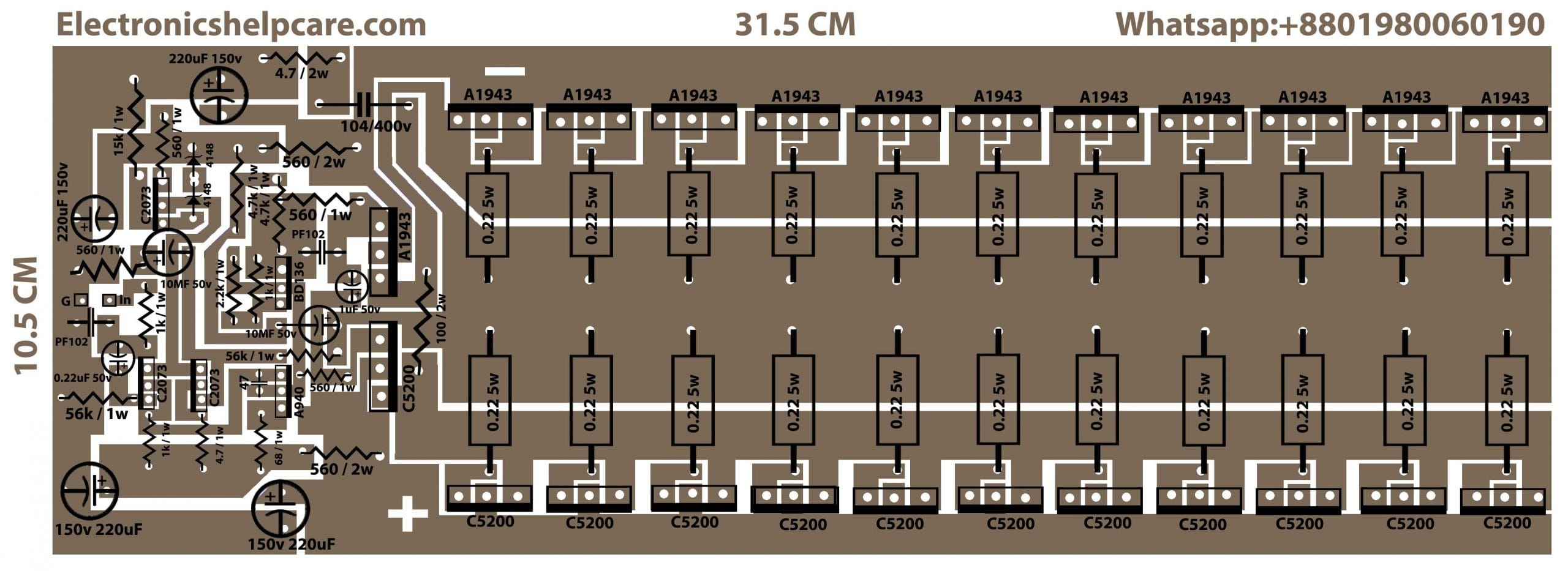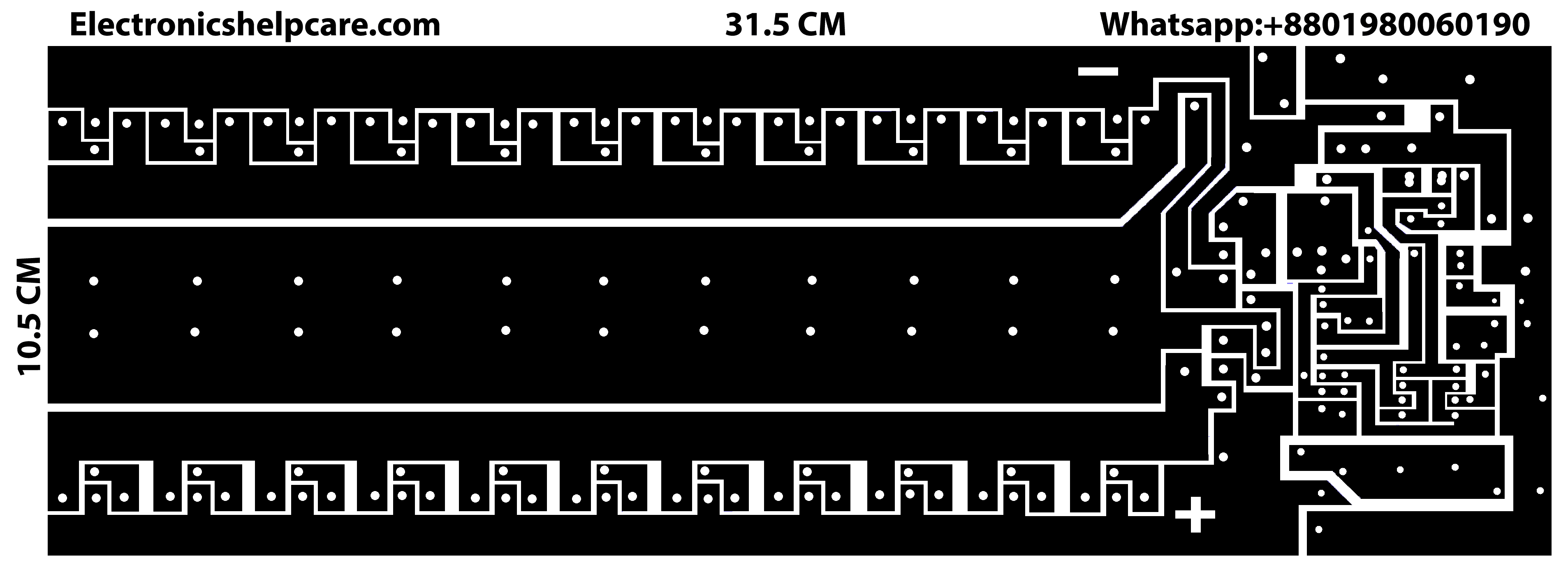Breaking News

# how to make 1000 watts amplifier?

## how to make 1000 watts amplifier:

transistor amplifier circuit diagram using 2sc5200 and 2sa1943. This is PNP and NPN amplifier circuit diagram. We used 2sc5200 and 2sa1943. 2sc5200 is an NPN transistor and 2sa1943 is a PNP transistor. We can use a maximum of 230 volts and 1.5 amperes. But here in this circuit diagram, we can use a maximum of 50 voltage. The voltage depends on various circuits. This circuit is for a maximum of 50 voltage. We can use a maximum of 1.5 amperes for 1 transistor.

transistor amplifier circuit diagram using 2sc5200 and 2sa1943Circuit diagram for this amplifiercircuit for printHow to calculate watts?

We know that voltage X ampere = watts, so if we use 2 transistors we have to use 35-0-35 voltage and 3 amperes because 1 transistor can take 1.5 amperes. According to watt, calculation rules 35 X 6=210 watts. Here we have a question why 6 amperes? Why not 3 amperes? Because here voltage is dual. According to the rules; voltage will be the same at 35 but ampere will be double 3+3=6. Our voltage starts from ground to 35 both sides positive and negative and both sides have 3 amp. In this circuit taking 35 volts and amperes from both sides are 3+3=6.

Using voltage and amperes for this 22 transistors circuit

In this circuit, we can use 50 voltage and 22 X 1.5=33 amperes maximum. But we use 50 voltage and 20 amperes for this circuit. So,50 X 20=1000 watts. We have to use 2 amplifier circuit diagrams for stereo sound. 1000+1000=2000 watts amplifier we are going to make.

transistor circuit diagram of 2sa1943 and 2sc5200

Thanks a lot to be with us. If you want another post then please visit our website.

We have another post for you. like repairing amplifier,